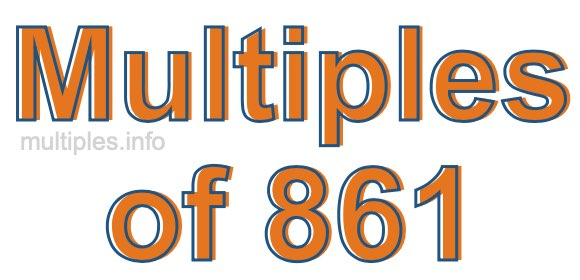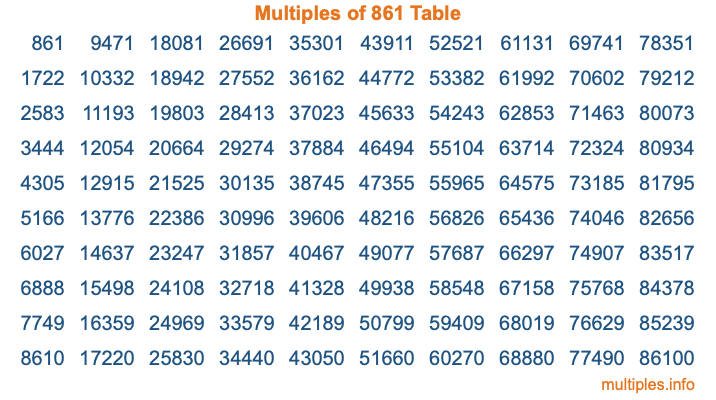Multiples of 861Welcome to the Multiples of 861 page. Here we will first teach you everything you will ever need to know about the multiples of 861, and then give you a study guide summary of everything we taught you to make sure you remember it all. Use this page to look up facts and learn information about the multiples of 861. This page will make you a multiples of eight hundred sixty-one expert!

Definition of Multiples of 861
Multiples of 861 are all the numbers that when divided by 861 equal an integer. Each of the multiples of 861 are called a multiple. A multiple of 861 is created by multiplying 861 by an integer.

Therefore, to create a list of multiples of 861, you start with 1 multiplied by 861, then 2 multiplied by 861, then 3 multiplied by 861, and so on for as long as you want. Thus, the list of the first five multiples of 861 is 861, 1722, 2583, 3444, and 4305. To see a larger list of multiples of 861, see the printable image of Multiples of 861 further down on this page. We also have a category where you can choose any nth multiple of 861.

Multiples of 861 Checker
The Multiples of 861 Checker below checks to see if any number of your choice is a multiple of 861. In other words, it checks to see if there is any number (integer) that when multiplied by 861 will equal your number. To do that, we divide your number by 861. If the the quotient is an integer, then your number is a multiple of 861.

Is  a multiple of 861?

Least Common Multiple of 861 and ...
A Least Common Multiple (LCM) is the lowest multiple that two or more numbers have in common. This is also called the smallest common multiple or lowest common multiple and is useful to know when you are adding our subtracting fractions. Enter one or more numbers below (861 is already entered) to find the LCM.

Check out our LCM Calculator if you need more details about the Least Common Multiple or if you need the LCM for different numbers for adding and subtraction fractions.

nth Multiple of 861
As we stated above, 861 is the first multiple of 861, 1722 is the second multiple of 861, 2583 is the third multiple of 861, and so on. Enter a number below to find the nth multiple of 861.

th multiple of 861

Multiples of 861 vs Factors of 861
861 is a multiple of 861 and a factor of 861, but that is where the similarities end. All postive multiples of 861 are 861 or greater than 861. All positive factors of 861 are 861 or less than 861.

Below is the beginning list of multiples of 861 and the factors of 861 so you can compare:

Multiples of 861: 861, 1722, 2583, 3444, 4305, etc.

Factors of 861: 1, 3, 7, 21, 41, 123, 287, 861

As you can see, the multiples of 861 are all the numbers that you can divide by 861 to get a whole number. The factors of 861, on the other hand, are all the whole numbers that you can multiply by another whole number to get 861.

It's also interesting to note that if a number (x) is a factor of 861, then 861 will also be a multiple of that number (x).

Multiples of 861 vs Divisors of 861
The divisors of 861 are all the integers that 861 can be divided by evenly. Below is a list of the divisors of 861.

Divisors of 861: 1, 3, 7, 21, 41, 123, 287, 861

The interesting thing to note here is that if you take any multiple of 861 and divide it by a divisor of 861, you will see that the quotient is an integer.

Multiples of 861 Table
Below is an image of the first 100 multiples of 861 in a table. The table is in chronological order, column by column. The first column has the first ten multiples of 861, the second column has the next ten multiples of 861, and so on.The Multiples of 861 Table is also referred to as the 861 Times Table or Times Table of 861. You are welcome to print out our table for your studies.

Negative Multiples of 861
Although not often discussed or needed in math, it is worth mentioning that you can make a list of negative multiples of 861 by multiplying 861 by -1, then by -2, then by -3, and so on, to get the following list of negative multiples of 861:

-861, -1722, -2583, -3444, -4305, etc.

Multiples of 861 Summary
Below is a summary of important Multiples of 861 facts that we have discussed on this page. To retain the knowledge on this page, we recommend that you read through the summary and explain to yourself or a study partner why they hold true.

There are an infinite number of multiples of 861.

A multiple of 861 divided by 861 will equal a whole number.

861 divided by a factor of 861 equals a divisor of 861.

The nth multiple of 861 is n times 861.

The largest factor of 861 is equal to the first positive multiple of 861.

861 is a multiple of every factor of 861.

861 is a multiple of 861.

A multiple of 861 divided by a divisor of 861 equals an integer.

861 divided by a divisor of 861 equals a factor of 861.

Any integer times 861 will equal a multiple of 861.

Multiples of a Number
Here you can get the multiples of another number, all with the same attention to detail as we did for multiples of 861 on this page.

Multiples of
Multiples of 862
Did you find our page about multiples of eight hundred sixty-one educational? Do you want more knowledge? Check out the multiples of the next number on our list!